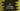# C program to find the third angle of a triangle if other two are given### C program to find the third angle of a triangle :

In this tutorial, we will learn how to find the third angle of a triangle if the first and second angle is given. We know that the sum of all three angles of a triangle is 180 degree. So, to find the third angle, we will subtract the sum of two angles from 180. Let’s take a look at the program :

### C Program code :

``````#include <stdio.h>
#define SUM 180

int main()
{
//1
float firstAngle, secondAngle, thirdAngle;

//2
printf("Enter first and second angle of the triangle : ");

scanf("%f %f", &firstAngle, &secondAngle);

//3
thirdAngle = SUM - (firstAngle + secondAngle);

//4
printf("You have entered %f and %f\n", firstAngle, secondAngle);
printf("Third Angle is : %f \n", thirdAngle);
}``````

### Explanation :

The commented numbers above indicate the steps number below :

1. First, create three float variables to store all three angles.
2. Ask the user to enter the first and second angle of the triangle. And store it in variables firstAngle and secondAngle.
3. Now, calculate the third angle and store the value in variable thirdAngle.
4. Print all angles: firstAngle,secondAngle and thirdAngle

### Sample Outputs :

``````Enter first and second angle of the triangle : 120 30
You have entered 120.000000 and 30.000000
Third Angle is : 30.000000

Enter first and second angle of the triangle : 20
30
You have entered 20.000000 and 30.000000
Third Angle is : 130.000000

Enter first and second angle of the triangle : 13.5
12.5
You have entered 13.500000 and 12.500000
Third Angle is : 154.000000
``````